# OECD Gross pension replacement rates data analysis 5 - t-test with simulation based using "infer" package of R. There is no significant difference between 2014 and 2020.Generated by Bing Image Creator

This post is following of the above post. In the above post, I visualize replacement rates of 2014 and 2020. And there seems no signficant difference.

In this post, let's confirm that using infer package of R.

First, let's get mean difference.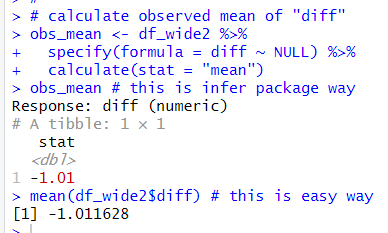I see the mean "diff" is -1.01, I use infer package workflow and usual mean() function.

Next, let's make null distribution, null hypothesis is mean "diff" = 0.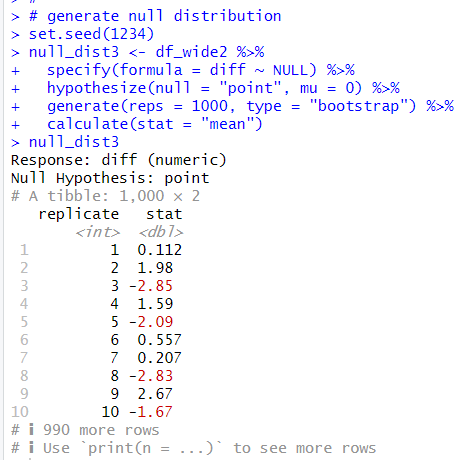Then, let's visualize p-value area.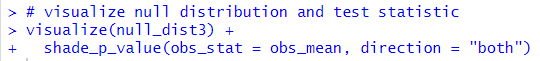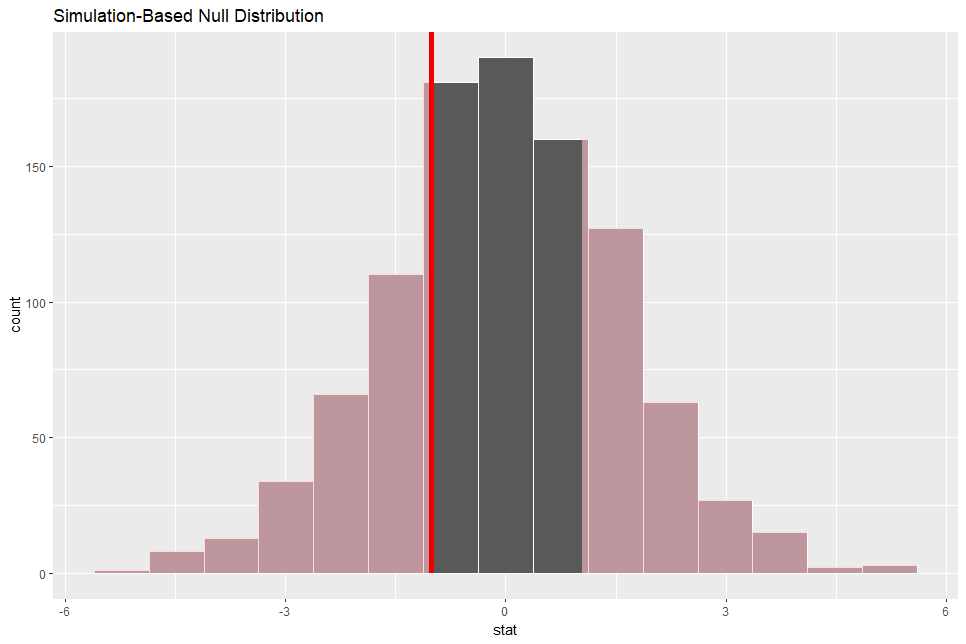red shaded are is p-value area, it seems very wide.

Now, let's get p-value.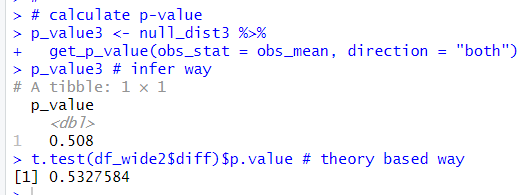p-value using infer workflow, which is simulation based, is 0.508. The theory based p-value, which is using t.test() function, is 0.508. The both p-value is greater than 0.5. I failed to reject null hypothesis: the mean difference = 0.

Next, let's make a bootstrap distribution.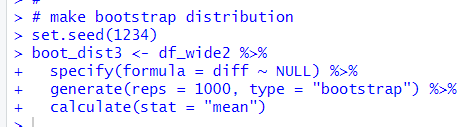Let's visualize it.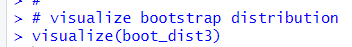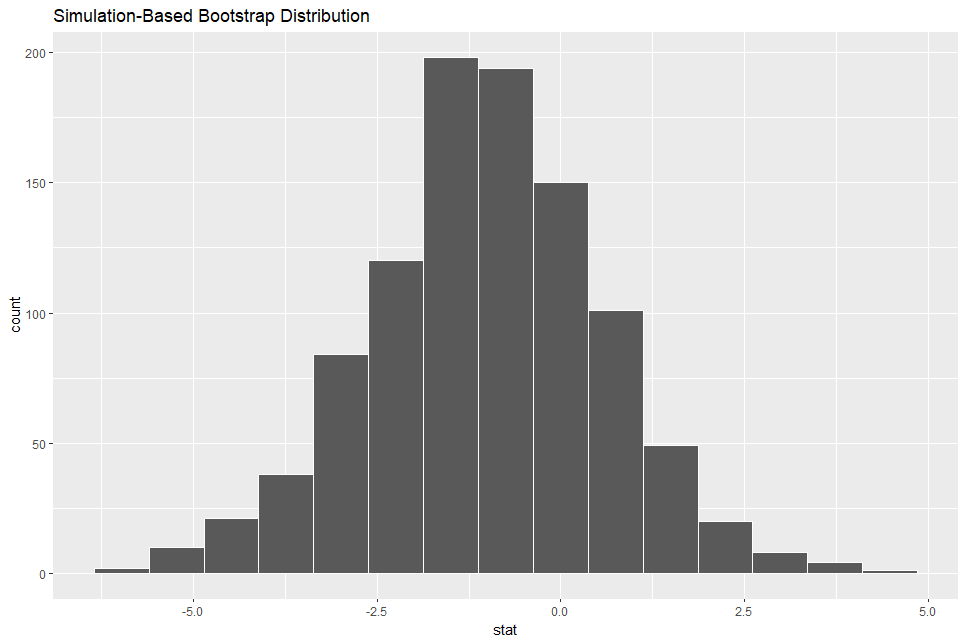The bootstrap distribution looks symmetric.

Let's calculate 95% confidence interval using percentile method.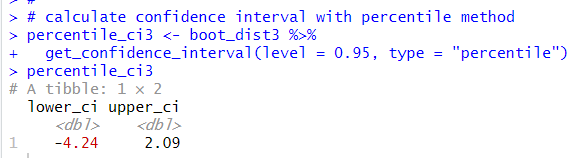95% confidence intervals is from -4.24 to 2.09. It includes 0, so, I cannot reject the null hypothesis.

I also use standard error method to calclate confidence interval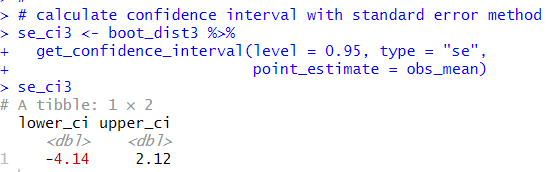The the standard error method confidence interval is from -4.14 to 2.12. It included 0 too.

And I use t.test() function to get theory based confidence interval.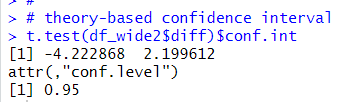The theory based confidence interval also includes 0.

Then, let's visualize confidence interval.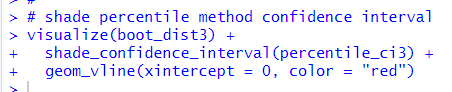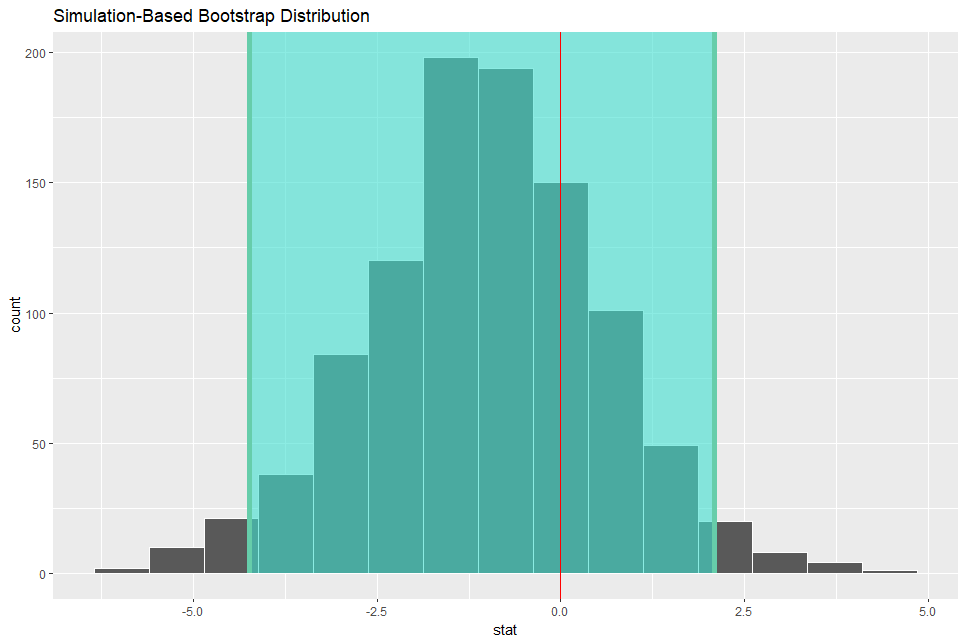I added red vertical line with geom_vline() function at x = 0.
So, I cannot reject the null hypothesis:the meand "diff" = 0.

So my cloncution is there is not statistically significant difference between 2014 and 2020.

That's it. Thank you!

Next post is

To read from the first posrt,